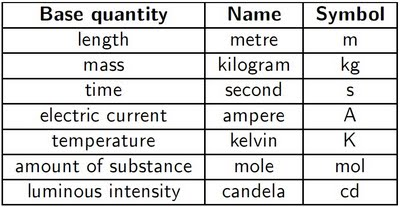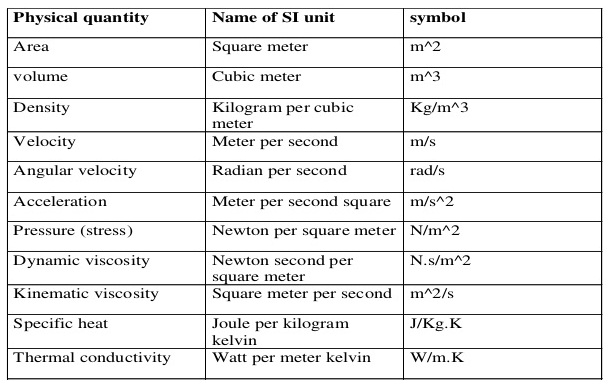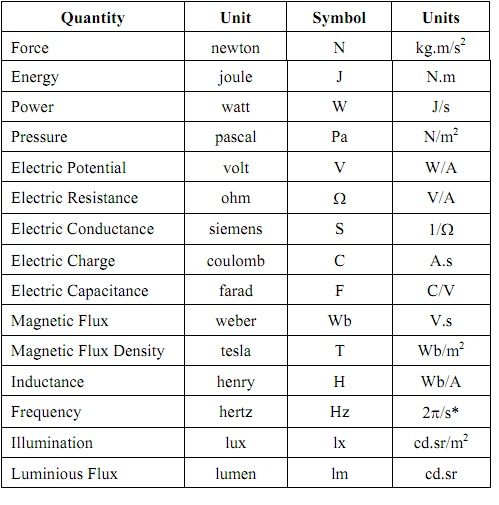#### Units and Dimensions, Chemistry tutorial

Units of Physical Quantities:

Concept of SI:

Till lately in the scientific world, mostly two systems of units have been in general use. One is the metric system that is, c.g.s (centimeter gram and second) which was more generally employed over the European continent and the other is f.p.s (foot pound and second) widespread in England. A common system of units assists in exchanging the scientific facts and ideas originating from various countries. It is better still if the system of units could be derived from the scientific formulae or fundamental constants.  This long felt require for a common system of scientific units was realized at a meeting known as General Conference on Weight and measures in the year  1960.  At this meeting, the international scientific community agreed to adopt common units of measurement termed as International System of Units. This is abbreviated as SI units from the French name Systeme Internationale.

Basic Units:

There are seven basic or fundamental physical quantities from which all the other physical quantities can be derived. Can you state two of those fundamental physical units you are familiar with? You are absolutely right if length, time, mass and temperature are the parts of what you stated in your answer. Each of such physical quantities consists of a unit termed basic unit having a specified symbol. The table illustrated below represents the names of these physical quantities all along with their symbols of SI units. Each of such quantities is regarded as having its own dimension. The dimensions of basic quantities are helpful in defining the derived physical quantities.

Table: Basic Physical Quantities and their UnitsBefore you go on, have you ever thought of what such basic quantities are? Supposing you can't remember what length, mass, time, temperature, electric current, luminous of intensity and amount of substance. There definitions are in brief stated here.

Remember that the length is a measure of distance or duration of time. Let us consider temperature as the measurement of the coldness or hotness of matter. Have you ever thought of the relationship between the temperature and heat? What is heat and what is temperature? What relationship exists among the two? We state that temperature signifies it is a property of matter whereas heat is an energy flow to and from matter. Whenever heat is added to matter or removed, there will be an increase or decrease in the temperature. The quantity of heat added to a matter find out its temperature. From the table, the unit of temperature is 'Kelvin' and not 'Celsius' or 'Fahrenheit'. Why is Kelvin utilized for temperature?

Though we are not going to define the kilogram, meter and so on. As the aim of this chapter is to utilize these units and not to establish the basis of such units. Have you ever think of how these units are employed in daily life experience? For illustration, a meter man comes home to read your meter, what does he read. The electric current or the quantity of electricity that will be employed to compute your bill. If your answer is electric current, why do you think you are correct?

Derived Units:

Apart from the basic physical quantities, there are others known as the derived physical quantities. As the name signifies, they are derived from the seven basic quantities illustrated in the table above. The derivations are completed by definitions comprising multiplication, division, differentiation and integration.

In the tables illustrated below, the derived SI units without and with special names are illustrated. We will find it helpful, all through our study of chemistry to refer to such tables whenever a few physical quantities are to be deduced. As electrochemistry will be studied, some of the helpful electrical quantities are as well comprised in the table below:

Table: Derived SI Units without special namesTable: Derived SI Units having special namesThe mathematical relationship to be employed for the ideal gas equation is as illustrated below:

Pressure x Volume = Amount of the substance x gas constant x temperature

Rearranging this,

R = (Pressure x Volume)/(Amount of the Substance x Temperature)

From the table, we make use of the dimensions of these quantities to derive the dimension and the unit of R as illustrated below:

Dimension of R = Dimension of [(Pressure x Volume)/(Amount of the Substance x Temperature)]

Dimension of R = PV/mT

Dimension of R = (Kg/m/s2 x m3)/(mole x K )

= (Kg.m2/s2) / (mole x K) as, 1 Joule = 1 Kg.m2/s2

Therefore R,

= J/mole. K

In general, the given hints would be helpful in the deduction of the unit of a quantity (which we name as the test quantity):

a) Write an equation associating the test quantity to other quantities of identified dimensions.

b)  Rearrange this equation in such a manner that merely the test quantity is on the left hand side and others are on the right hand side.

c)  Replace the dimensions of the quantities on the right hand side and simplify.

d) Write down the units corresponding to the simplified dimensions, by using the above three tables.

Tutorsglobe: A way to secure high grade in your curriculum (Online Tutoring)